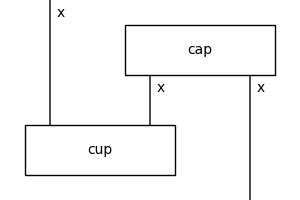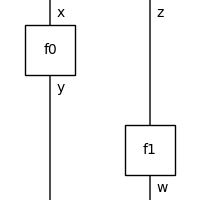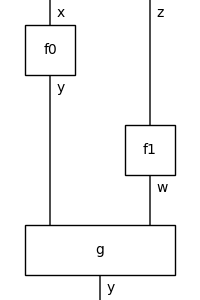# Diagram#

class discopy.monoidal.Diagram(inside, dom, cod, _scan=True)[source]#

A diagram is a tuple of composable layers `inside` with a pair of types `dom` and `cod` as domain and codomain.

Parameters:
• inside (tuple[Layer, ...]) – The layers of the diagram.

• dom (T) – The domain of the diagram, i.e. its input.

• cod (T) – The codomain of the diagram, i.e. its output.

Summary

 `tensor`([other]) Parallel composition, called using `@`. `boxes` The boxes in each layer of the diagram. `offsets` The offset of a box is the length of the type on its left. `draw`(**params) Draws a diagram using networkx and matplotlib. `interchange`(i, j[, left]) Interchange a box from layer `i` to layer `j`. `normalize`([left]) Implements normalisation of boundary-connected diagrams, see Delpeuch and Vicary Delpeuch and Vicary [DV22]. `normal_form`(**params) Returns the normal form of a diagram.
ty_factory#

alias of `Ty`

layer_factory#

alias of `Layer`

classmethod from_callable(dom, cod)[source]#

Define a diagram using the standard syntax for Python functions.

Note that we can specify the offset as argument.

Example

```>>> x = Ty('x')
>>> cup, cap = Box('cup', x @ x, Ty()), Box('cap', Ty(), x @ x)
>>> @Diagram.from_callable(x, x)
... def snake(left):
...     middle, right = cap(offset=1)
...     cup(left, middle)
...     return right
>>> snake.draw(
...     figsize=(3, 3), path='docs/_static/drawing/diagramize.png')
```Parameters:
• dom (Ty) –

• cod (Ty) –

Return type:

Callable[[Callable], Diagram]

tensor(other=None, *others)[source]#

Parallel composition, called using `@`.

Parameters:
• other (Optional[Diagram]) – The other diagram to tensor.

• rest – More diagrams to tensor.

• others (Diagram) –

Return type:

Diagram

Important

The definition of tensor is biased to the left, i.e.:

```self @ other == self @ other.dom >> self.cod @ other
```

Example

```>>> x, y, z, w = Ty('x'), Ty('y'), Ty('z'), Ty('w')
>>> f0, f1 = Box('f0', x, y), Box('f1', z, w)
>>> assert f0 @ f1 == f0.tensor(f1) == f0 @ Id(z) >> Id(y) @ f1
```
```>>> (f0 @ f1).draw(
...     figsize=(2, 2),
...     path='docs/_static/monoidal/tensor-example.png')
```property boxes: list[discopy.monoidal.Box]#

The boxes in each layer of the diagram.

property offsets: list[int]#

The offset of a box is the length of the type on its left.

property width#

The width of a diagram, i.e. the maximum number of parallel wires.

Example

```>>> x = Ty('x')
>>> f = Box('f', x, x ** 4)
>>> diagram = f @ x ** 2 >> x ** 2 @ f.dagger()
>>> assert diagram.width == 6
```
encode()[source]#

Compact encoding of a diagram as a tuple of boxes and offsets.

Example

```>>> x, y, z, w = Ty('x'), Ty('y'), Ty('z'), Ty('w')
>>> f0, f1, g = Box('f0', x, y), Box('f1', z, w), Box('g', y @ w, y)
>>> diagram = f0 @ f1 >> g
>>> dom, boxes_and_offsets = diagram.encode()
>>> assert dom == x @ z
>>> assert boxes_and_offsets == [(f0, 0), (f1, 1), (g, 0)]
>>> assert diagram == Diagram.decode(*diagram.encode())
>>> diagram.draw(figsize=(2, 2),
...        path='docs/_static/monoidal/arrow-example.png')
```Return type:

tuple[discopy.monoidal.Ty, list[tuple[discopy.monoidal.Box, int]]]

classmethod decode(dom, boxes_and_offsets=None, boxes=None, offsets=None, cod=None)[source]#

Turn a tuple of boxes and offsets into a diagram.

Parameters:
• dom (Ty) – The domain of the diagram.

• cod (Optional[Ty]) – The codomain of the diagram.

• boxes_and_offsets (Optional[list[tuple[discopy.monoidal.Box, int]]]) – The boxes and offsets of the diagram.

• boxes (Optional[list[discopy.monoidal.Box]]) – The list of boxes.

• offsets (Optional[list[int]]) – The list of offsets.

Return type:

Diagram

Example

```>>> x, y, z, w = map(Ty, "xyzw")
>>> f, g = Box('f', x, y), Box('g', z, w)
>>> assert f @ z >> y @ g == Diagram.decode(
...     dom=x @ z, cod=y @ w, boxes=[f, g], offsets=[0, 1])
```

Note

If `boxes_and_offsets is None` then we set it to `zip(boxes, offstes)`.

to_drawing()[source]#

Called before `Diagram.draw()`.

to_staircases()[source]#

Splits layers with more than one box into staircases.

Example

```>>> x, y = Ty('x'), Ty('y')
>>> f0, f1 = Box('f0', x, y), Box('f1', y, x)
>>> diagram = y @ f0 >> f1 @ y
>>> print(diagram.foliation())
f1 @ f0
>>> print(diagram.foliation().to_staircases())
f1 @ x >> x @ f0
```
foliation()[source]#

Merges layers together to reduce the length of a diagram.

Example

```>>> from discopy.monoidal import *
>>> x, y = Ty('x'), Ty('y')
>>> f0, f1 = Box('f0', x, y), Box('f1', y, x)
>>> diagram = f0 @ Id(y) >> f0.dagger() @ f1
>>> print(diagram)
f0 @ y >> f0[::-1] @ y >> x @ f1
>>> print(diagram.foliation())
f0 @ y >> f0[::-1] @ f1
```

Note

If one defines a foliation as a sequence of unmergeable layers, there may exist many distinct foliations for the same diagram. This method scans top to bottom and merges layers eagerly.

depth()[source]#

Computes (an upper bound to) the depth of a diagram by foliating it.

Example

```>>> x, y = Ty('x'), Ty('y')
>>> f, g = Box('f', x, y), Box('g', y, x)
>>> assert Id(x @ y).depth() == 0
>>> assert f.depth() == 1
>>> assert (f @ g).depth() == 1
>>> assert (f >> g).depth() == 2
```

Note

The depth of a diagram is the minimum length over all its foliations, this method just returns the length of `Diagram.foliation()`.

interchange(i, j, left=False)[source]#

Interchange a box from layer `i` to layer `j`.

Parameters:
• i (int) – Index of the box to interchange.

• j (int) – Index of the new position for the box.

• left – Whether to apply left interchangers.

Return type:

Diagram

Note

By default, we apply right interchangers:

```top >> left @ box1.dom @ mid @ box0     @ right\
>> left @ box1     @ mid @ box0.cod @ right >> bottom
```

gets rewritten to:

```top >> left @ box1     @ mid @ box0.dom @ right\
>> left @ box1.cod @ mid @ box0     @ right >> bottom
```
normalize(left=False)[source]#

Implements normalisation of boundary-connected diagrams, see Delpeuch and Vicary Delpeuch and Vicary [DV22].

Parameters:

left – Passed to `Diagram.interchange()`.

Return type:

Iterator[Diagram]

Example

```>>> from discopy.monoidal import *
>>> s0, s1 = Box('s0', Ty(), Ty()), Box('s1', Ty(), Ty())
>>> gen = (s0 @ s1).normalize()
>>> for _ in range(3): print(next(gen))
s1 >> s0
s0 >> s1
s1 >> s0
```
normal_form(**params)[source]#

Returns the normal form of a diagram.

params : Passed to `Diagram.normalize()`.

Raises:

NotImplementedError – Whenever `normalize` yields the same rewrite steps twice, e.g. the diagram is not boundary-connected.

Return type:

Diagram

bubble_factory#

alias of `Bubble`

draw(**params)#

Draws a diagram using networkx and matplotlib.

Parameters:
• draw_as_nodes (bool, optional) – Whether to draw boxes as nodes, default is `False`.

• color (string, optional) – Color of the box or node, default is white (`'#ffffff'`) for boxes and red (`'#ff0000'`) for nodes.

• textpad (pair of floats, optional) – Padding between text and wires, default is `(0.1, 0.1)`.

• draw_type_labels (bool, optional) – Whether to draw type labels, default is `False`.

• draw_box_labels (bool, optional) – Whether to draw box labels, default is `True`.

• aspect (string, optional) – Aspect ratio, one of `['auto', 'equal']`.

• margins (tuple, optional) – Margins, default is `(0.05, 0.05)`.

• nodesize (float, optional) – Node size for spiders and controlled gates.

• fontsize (int, optional) – Font size for the boxes, default is `12`.

• fontsize_types (int, optional) – Font size for the types, default is `12`.

• figsize (tuple, optional) – Figure size.

• path (str, optional) – Where to save the image, if None we call `plt.show()`.

• to_tikz (bool, optional) – Whether to output tikz code instead of matplotlib.

• asymmetry (float, optional) – Make a box and its dagger mirror images, default is `.25 * any(box.is_dagger for box in diagram.boxes)`.

factory#

alias of `Diagram`

sum_factory#

alias of `Sum`

to_gif(*diagrams, **params)#

Builds a gif with the normalisation steps.

Parameters:
• diagrams (`Diagram`, optional) – Sequence of diagrams to draw.

• path (str) – Where to save the image, if `None` a gif gets created.

• timestep (int, optional) – Time step in milliseconds, default is `500`.

• loop (bool, optional) – Whether to loop, default is `False`

• params (any, optional) – Passed to `Diagram.draw()`.

to_grid()#

Layout a diagram on a grid.

The first row is a list of `Wire` cells, then for each layer of the diagram there are two rows: the first for the boxes and the wires in between them, the second is a list of `Wire` for the outputs.

Parameters:

diagram (monoidal.Diagram) – The diagram to layout on a grid.

Return type:

Grid

```>>> from discopy.monoidal import *
>>> x = Ty('x')
>>> f, s = Box('f', x, x @ x), Box('s', Ty(), Ty())
>>> diagram = (
...     f @ f[::-1] >> x @ s @ x @ x >> f @ f[::-1]).foliation()
>>> print(diagram.to_grid())
Grid([Wire(1, x), Wire(15, x), Wire(17, x)],
[Cell(0, 12, f), Cell(14, 18, f[::-1])],
[Wire(1, x), Wire(11, x), Wire(15, x)],
[Cell(0, 4, f), Cell(6, 8, s), Cell(10, 16, f[::-1])],
[Wire(1, x), Wire(3, x), Wire(11, x)])
```